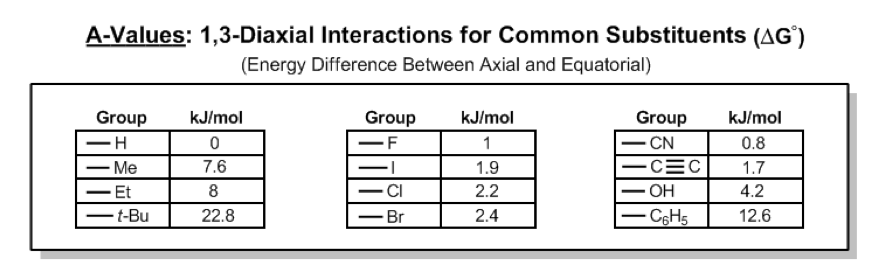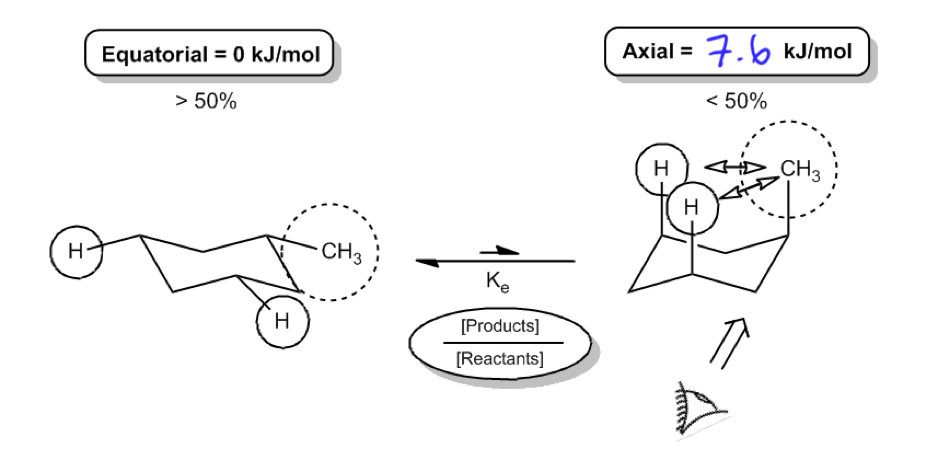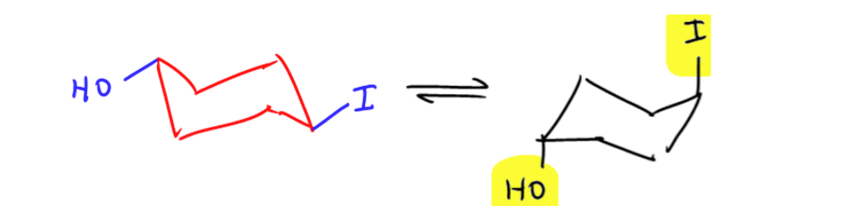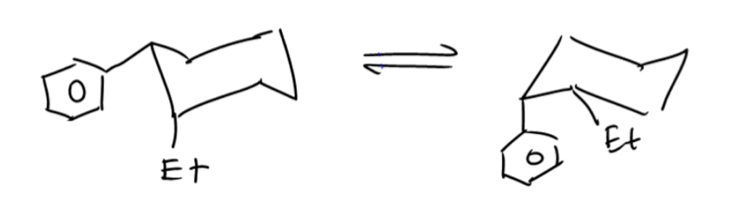Clutch Prep is now a part of Pearson
Ch. 4 - Alkanes and CycloalkanesWorksheetSee all chapters

# Calculating Energy Difference Between Chair Conformations

See all sections
Sections
IUPAC Naming
Alkyl Groups
Naming Cycloalkanes
Naming Bicyclic Compounds
Naming Alkyl Halides
Naming Alkenes
Naming Alcohols
Naming Amines
Cis vs Trans
Conformational Isomers
Newman Projections
Drawing Newman Projections
Barrier To Rotation
Ring Strain
Axial vs Equatorial
Cis vs Trans Conformations
Equatorial Preference
Chair Flip
Calculating Energy Difference Between Chair Conformations
A-Values
Decalin
Alkyl
t-Butyl, sec-Butyl, isobutyl, n-butyl

For most classes all you will need to know how to do is use equatorial preference to predict the most stable chair conformation.

However, sometimes you will be required to use energetics to calculate the exact percentages of each chair in solution. This is a multistep process, so here I’m going to walk you through it from scratch.

###### Calculating Flip Energy

First we have to introduce the concept of an A-value, which is simply the energy difference between the equatorial (most stable) and axial (least stable) positions.

Concept #1: Explaining how A-Values are related to cyclohexane flip energy

Transcript

All right guys, so in the next few videos I'm going to be going over a much more rigorous description of conformational analysis. Now the reason that we've been recording these to begin with is because your textbook goes through all this detail and I'm just trying to be as comprehensive as possible.
But this also happens to be an area of organic chemistry that many professors don't teach because they just find it too mathematical and too tedious. So you're going to be responsible to talk to your professor or look at old exams or whatever and determine if this is something that you need to know or not. I'm just teaching it just in case.
Now as a side note, I'm going to need you to – since it is mathematical, drop your iPhone calculator or whatever and grab one of these because we're actually going to be doing equations with e and logarithms and stuff like that. So if you need to pause the video to grab this, by all means, go for it. Let's go right into it.
The first thing that we're going to do is learn how to calculate flip energy. This has to do with cyclohexanes. Sometimes we're going to be required to actually calculate these numbers in kilojoules per mole and calculate the expense that it takes for a molecule to flip from equatorial to axial. Remember that I told you guys that equatorial preference states that you're always trying to be in that more stable position.
But how much energy does it actually take for the molecule to flip into that less stable position? Well, thankfully, scientists have done those scientific experiments for us and they developed something that we call A values. This term, A values, is a term that you're not going to find in your textbook, but it is found in more complicated texts and obviously if you look online, you would see that this is called A values. So you can Wikipedia it later.
Basically what is an A value? All it is is it's the sum of all of the energy expenses of the 1,3-diaxial interactions that are created by going into the axial position. Essentially, in those parentheses, what I have is it's literally just the energy difference between the axial and equatorial positions in kilojoules per mole.
Now whenever you're measuring anything with energy, you have to be aware that some texts and some professors like to use kilojoules per mole and some professors like to use kilocalories per mole. Now I'm just going to lower the screen for just one second so you can that there is just a really easy conversion here. Remember that 1 kilocal per mole equals 4.184 kilojoules per mole. So anything that I'm teaching you today in kilojoules you can apply to kilocals, you just have to do that simple dimensional analysis.
Now personally, I'm going to choose to do everything in kilocalories per – I'm sorry, exact opposite, kilojoules per mole. The reason is because all of the equations that we're going to use in the next few videos are in kilojoules, so it doesn't make sense to go back and forth between kilocalories. That's just a waste of time.
So now here are some of the most important A values in kilojoules per mole and these are not for you to memorize, but just you can see as a general trend that as your groups get bigger, the values get larger as well.
So you can see that – let's just look at an easy definition here. Hydrogen has an A value of 0. Could you describe or kind of explain why it would have an A value of 0? Because, guys, if you're substituent is hydrogen, all of the subsituents are hydrogen and that means there's no difference between axial and equatorial. That means that when you flip it, it's the same exact energy. So hydrogen is our standard. That basically means that there's zero energy lost or it costs zero energy to have it in one position versus another.
Now if we all of the sudden make it into a methyl group, now you can see the A value goes up significantly because now what that's saying is that it costs 7.6 kilojoules per mole to have a cyclohexane rest in the axial position versus the equatorial position. So you're going to be expending 7.6 kilojoules per mole to keep it in that unstable position.
As you can see, as the groups get bigger, ethyl, tertbutyl, these values start to get really crazy high. Just so you guys know, 23 kilojoules per mole is a large number in organic chemistry.
As you can see I've got the halogens. Interestingly, the halogens don't change much. Notice that iodine, chlorine, and bromine are all about the same and they're not really in the right order that you would expect. You would think that maybe as you get bigger, the harder it's going to be to switch it to the axial position, but remember halogens are unique because their bond lengths also get longer.
Look at iodine. It's in an interesting position where it happens to be the biggest one, so you would think oh heck, that thing does not want to move axial, but it also has the longest bond length, so it doesn't really have a lot of interactions with the hydrogens that are next to it.
Really quick, I know I'm kind of jumping around, but this is exactly a diagram of those 1,3-diaxial interactions. Imagine that – I'm going to erase this – but image that this is my target molecule. Imagine that that's my iodine or whatever. The 1,3-diaxial interactions are the interactions experienced with the hydrogens that are also axial, correct? Well, what I'm trying to say is that iodine, for example, has a super long bond, so it's almost out of the way from those hydrogens. So just kind of an exception. Again, please don't memorize it. It's just interesting.
Then we've got some other weird substituents that I just thought were interesting and I thought might be relevant. So I have a cyanide. I have an alkyne alcohol, very important. And then a phenyl group – a phenol group. I'm sorry, a phenol group.
Let's move ahead to this diagram here. So here I have my equatorial position. As you can see, I'm just looking at an example of methylcyclohexane. It's in the equatorial position. Now it's going to take energy for it move to the axial position. How much energy? Well, for that we'd have to look at our A values and our A values say 7.6. That's what I would write here. That the axial is going to cost me 7.6 kilojoules per mole.
Now according to what we learned in the past, we would have easily been able to say that over 50% of the molecule is going to be equatorial and less that 50% is going to be axial. Why? Because we know that equatorial preference states that you favor the equatorial side, so favor means that more than half is that and less than half is this. Perfect. But in this exercise, by the end of these videos, we're going to be able to calculate the exact percentages, not just this one's better or this one's worse. I'm going to be able to say that 95% of it is going to be equatorial and only 5% will be axial. How do we get there? Through these calculations.
Now I just have to go over a few more terms. Remember that Ke is your equilibrium constant. It's defined by the products over the reactants. So I would expect that my Ke would go to the left in this case, that my Ke would have a value that would favor the equilibrium, the equatorial molecule.
Now remember that Ke is products over reactants. That's what it's defined as, so when we do calculations with Ke later, we're always going to use this definition of products over reactants, where products is your axial and reactants is your equatorial. Now you might be asking me, “Johnny, why is products axial?” Because that's the thing we're trying to make. We're trying to get it to go axial. So I'm saying what amount of this is going to go to the axial position, what amount of this is going to go to the equatorial position. Perfect
So now I want to just make a quick note here guys of that 7.6 number. It seemed random, but it's actually related to something that we've learned in the past because if you took let's say an eyeball, let's say you're trying to make a Newman projection, and you were trying to see how this bond looked, what you would actually find is that the hydrogens are actually in a gauche conformation to the methyl group.
So see here, I've got this methyl group. And then I've got this hydrogen that's coming off of the gauche position. Do you guys recall what was the energy that it took to move a hydrogen into the gauche position for a methyl group? It was actually 3.8 kilojoules per mole. Now you can't see it in this diagram because I'm kind of splitting it down the middle, but you would have the same exact gauche conformation over here. You'd have the same exact gauche interaction over there. It's just you have to rotate the molecule.
What's crazy is that these 1,3-diaxial interactions are just accumulations of these gauche interactions that we had talked about for Newman projections. So a gauche conformation costs 3.8 for a methyl and a hydrogen. Well what if you have two of them? Then that's going to equal your A value. So an A value is really just a sum of these gauche interactions that are happening on a Newman projection.
Kind of interesting. Again, you don't need to calculate this. I just find it interesting that you can actually somewhat derive or approximate these A values just from learning your conformational values from Newman projections, so these concepts are related.We can use these values to calculate how much energy it is going to take to flip a chair into its least stable form.Note: The above chair flip in the video is slightly off. Remember that the direction of the groups (up vs. down) should not change when going from axial to equatorial or vice versa.

All the math is still correct here, but I should have drawn the groups down instead of up on the second chair. :)

[Refer to the videos below for examples of this]

Practice: Calculate the difference in Gibbs free energy between the alternative chair conformations of trans-4-iodo-1-cyclohexanol.Practice: Calculate the difference in Gibbs free energy between the alternative chair conformations of cis-2-ethyl-1-phenylcyclohexane.